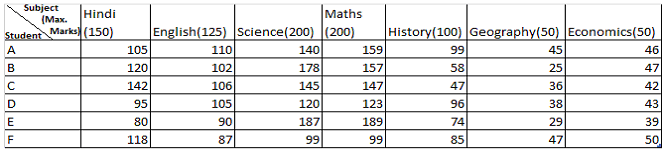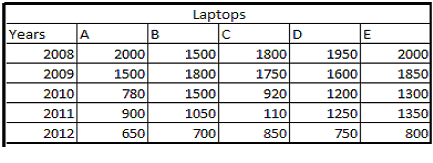# IBPS Quantitative Aptitude model test paper - 4

Home > Aptitude test > Quantitative model test - 4

## IBPS Quantitative Aptitude model test paper - 4

Directions (Q.1-5) What value should come in place of the question mark (?) in the following question?

Q1. √3025 + √2025 = ?

A. 10
B. 100
C. 152
D. 110

Q2. 1892 ÷ 31 – 121 = ?

A. 60
B. -59
C. 96
D. 19

Q3. 1201.964 – (0.121 x 0.231 x 0.191) = ?

A. 1201.959
B. 1200
C. 1199.036
D. 1036.36

Q4. 12.5% of 550 - 11% of 221 = ? – 1.56

A. 66
B. 46
C. 16
D. 106

Q5. 3/5 of 1/3 of 1/4 of 8000 = ?

A. 450
B. 400
C. 480
D. 425

Q6. 24 employees can develop a software in forty days. How many employees are required to develop the same software in 20 days?

A. 48
B. 12
C. 40
D. 42

Q7. Find the compound interest on the sum of Rs. 4000@ 12%p.annum at the end of two years ?

A. 1018.50
B. 1017.60
C. 1018
D. 1020.50

Q8. In how many ways can the letters of the word ‘ABCDEF’ be arranged?

A. 1240
B. 1440
C. 720
D. 360

Q9. What will come in place of question mark (?) in the following number series?

248 ? 284 299 312.

A. 280
B. 250
C. 267
D. 275

Q10. Find the sum of second largest and the smallest number, if the sum of five consecutive odd numbers is 595?

A. 236
B. 351
C. 263
D. 298

Q11. The following questions are accompanied three statement a, b and c. You have to determine which statement(s) is /are necessary sufficient to answer the question?

In how many days can the work be done by thirteen boys and twentysix girls ?

a. 8 boys and 21 girls can complete the work in 1 days.
b. 4 boys and 4 girls can complete the work in 3 days.
c. 12 boys and 20 girls can complete the work in 5 days.

A. Only a.
B. Only b.
C. Only c.
D. None of these.

Directions (Q. 12-16) The following table gives the percentage of marks obtained by seven students in seven different subjects in an examination. Study the table and answer the question

based on it.Q12. What was the aggregate of marks obtained by student ‘C’ in all the seven subjects ?

A. 665
B. 506
C. 589
D. 575

Q13. What is the overall percentage of student ‘B’ ?

A. 78.51
B. 76
C. 80
D. 70

Q14. What are the average marks obtained by all the six students in Physics?

A. 650
B. 756
C. 658
D. 815

Q15. The number of students who obtained 80% and above marks in all the subjects is :

A. One
B. Two
C. Three
D. All students

Q16. Which student secured maximum marks among all the students ?

A. B
B. D
C. A
D. F

Directions (Q. 17-18). In the following questions two equations numbered I and II are given. You have to solve both the equations and give answer

1) If a> b
2) If a>= b
3) If a < b
4) If a=5) If a= b or the relationship cannot be established

Q17. I. a1/2 - 61/2 / a1/2 = 0
II. b3 = 6 3/2

A. Only 1 follows
B. Only 2 follows
C. Either 1 or 2 follows
D. Only 5 follows.

Q18. I. (a+6) 1/2 = 1211/2 – 361/2
II. b2 + 112 = 473

A. Only 1 follows
B. Only 2 follows
C. Only 4 follows
D. Only 5 follows.

Directions (Q.19-23) . Study the following table carefully and answer the questions given below:

Number of five types of Laptops manufactured by a company over the years (in thousand).Q19. In the given years which type of total manufactured laptops were maximum?

A. C
B. D
C. E
D. A

Q20. What was the percentage drop in manufacture of ‘A’ type Laptops from 2008 to 2010 ?

A. 61%
B. 36%
C. 48%
D. 54%

Q21. Which type of Laptop there was a continuous increase in manufacture over the years?

A. B
B. D
C. E
D. None of these

Q22. The manufacture of E type laptops in 2010 was what percent of manufacture of B type laptops in 2008?

A. 75%
B. 66.66%
C. 86.66%
D. None of these

Q23. What was the approximate percentage increase in manufacture of A type toys from 2010 to 2011.

A. 13.33%
B. 6.7%
C. 12.25%
D. 24.33%

Q24. What will be the difference between S.I. and Compound on Rs.1500 for one year at 10% p.a. reckoned half-yearly is:

A. 3.75
B. 7.00
C. 12.25
D. 53.75

Q25. There are 8 Orange, 6 White and 7 Black pens in a Parul Bag. Parul picked up randomly a pen. What is the probability that it is neither orange nor white?

A. 1/6
B. 7/3
C. 21/14
D. 1/3